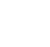How to calculate 'breakeven'

Plugging numbers into an equation you don’t understand may lead you to the wrong direction.
By Doug and Polly White |Breakeven will help you know how much your sales have to grow before you can reach profitability. With this information, you can estimate how long you will need to sustain losses, and to plan your cash flow accordingly.

Related: Understanding the Difference between Gross Margin and Markup

We could just give you a formula for calculating breakeven. Unfortunately, though, plugging numbers into an equation you do not understand may yield answers that will lead you to the wrong direction. Understanding only a few details can help you avoid that pitfall.

How to calculate breakeven

In its simplest terms, breakeven occurs when your business is neither making nor losing money.

In other words,

Revenue = total expenses

For example, think about two types of expenses: fixed costs and variable costs. Fixed costs are those that do not change as the number of units you sell grows. For example, if you are a retail store, rent is likely to be the same regardless of the number of units sold.

On the other hand, variable costs are those that increase as the number of units you sell grows. Again, if you are a retail store, when the number of units increases, the money you pay for the merchandise you sell will also increase. These costs vary with the number of units sold.

Therefore:

Total expense = variable costs + fixed costs

Variable costs may be expressed as the number of units sold times the variable cost per unit.

Therefore,

Total expense = (units x variable cost per unit) + fixed costs

Related: Cutting Your Price Has No End But Adding Value Has No Limits

Obviously, the variable cost per unit will not likely be the same for every unit you sell. Some items will be more expensive; others will cost less. Therefore, the variable cost per unit will be an average. Total revenue may be expressed as price times units sold. Therefore, at breakeven:

Price x units = (units x variable cost per unit) + fixed costs

Solving for the number of Units that needs to be sold to breakeven yields

Breakeven units = fixed costs/(price – variable cost per unit)

This makes sense. Price minus variable cost per unit is the amount of money you have to cover fixed costs each time you sell a unit. Let us say that fixed costs are P2,000 per month while the average price for the things you sell is P2, and the average variable cost per unit is P1.

This means that every time you sell a unit, you get P1 toward covering fixed costs. Because fixed costs are P2,000 per month, you will need to sell 2,000 units each month to achieve breakeven.

Obviously, once you calculate breakeven units, this figure can be converted into breakeven revenue. In our example above, the breakeven units number is 2,000, but each unit sells for P2. Therefore, breakeven revenue is P4,000 (2,000 x \$2). It is also possible to calculate breakeven revenue directly without calculating breakeven units first. To do this, multiply both sides of the equation above by price.

Price x breakeven units = fixed costs x (price/(price – variable cost per unit)

However,

(Price – variable cost per unit)/price = gross margin percentage

Therefore,

Price/(price – variable cost per unit) = 1/ gross margin percentage

Further,

Breakeven revenue = price x breakeven units

Plugging this into the equation above yields:

Breakeven revenue = fixed costs x (1/gross margin percentage)

or,

Breakeven revenue = fixed costs/gross margin percentage

One word of caution: Make sure that you understand what costs are really fixed, given the revenue change you are contemplating. Costs that are fixed for a 10% or 20% increase in revenue may not be fixed if the business has to grow by a factor of five.

Make sure you understand how your cost structure will change over the range of growth you need to reach breakeven. Failure to do so can result in grossly underestimating the breakeven, which can result in very bad business decisions.

Related: 3 Lessons About Setting Your Price Learned From a Vegas Prostitute

Understanding your business’ breakeven point is powerful, but you will need to ensure you fully understand the economics of the challenge you face. Basing business decisions on an oversimplified analysis can lead to disaster. Getting a good grip on the details outlined above will enable you to avoid this problem.

*****

Close